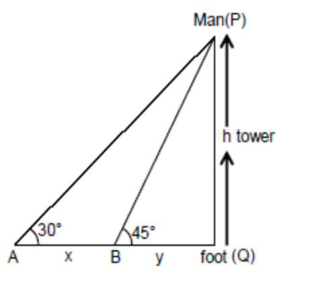# A man is observing, from the top of a tower,Question:

A man is observing, from the top of a tower, a boat speeding towards the tower from a certain point $\mathrm{A}$, with uniform speed. At that point, angle of depression of the boat with the man's eye is $30^{\circ}$ (Ignore man's height). After sailing for 20 seconds towards the base of the tower (which is at the level of water), the boat has reached a point $\mathrm{B}$, where the angle of depression is $45^{\circ}$. Then the time taken (in seconds) by the boat from B to reach the base of the tower is :

1. (1) $10(\sqrt{3}-1)$

2. (2) $10 \sqrt{3}$

3. (3) 10

4. (4) $10(\sqrt{3}+1)$

Correct Option: , 4

Solution:$\frac{\mathrm{h}}{\mathrm{x}+\mathrm{y}}=\tan 30^{\circ}$

$x+y=\sqrt{3} h$

Also

$\frac{\mathrm{h}}{\mathrm{y}}=\tan 45^{\circ}$

$\mathrm{h}=\mathrm{y}$

put in (1) $x+y=\sqrt{3} y$

$x=(\sqrt{3}-1) y$

$\frac{\mathrm{x}}{20}=^{\prime} \mathrm{v}^{\prime}$ speed

$\therefore$ time taken to reach

Foot from B

$\Rightarrow \frac{\mathrm{y}}{\mathrm{V}}$

$\Rightarrow \frac{x}{(\sqrt{3}-1) \cdot x} \times 20$

$\Rightarrow 10(\sqrt{3}+1)$